﻿ 基于有限体积法对洪水汇合流流场的数值模拟

# 基于有限体积法对洪水汇合流流场的数值模拟Numerical Simulation of Flood Confluence Flow Field Based on Finite Volume Method

Abstract: In this study, the finite volume method was used to simulate and analyze the complex flow field distribution of flood discharge and flood discharge project, and the confluence flow field near the flood gate was analyzed. After the flow field changes, the flow field distribution characteristics and flow velocity parameters are proposed. The influence of confluent flow field on the flood storage project buildings and nearby levees is analyzed. The hydraulic indexes near the flood control area and the flood diversion area are proposed, which are the flood gate layout and dam. Protection provides a scientific basis.

Abstract:

Abstract:

1. 引言

2. 数学模型

2.1. 控制方程

$\frac{\partial U}{\partial t}+\frac{\partial F}{\partial x}+\frac{\partial G}{\partial y}=S$ (1)

$U=\left(\begin{array}{c}H\\ {q}_{x}\\ {q}_{y}\end{array}\right)，\text{\hspace{0.17em}}F=\left(\begin{array}{c}{q}_{x}\\ \frac{{q}_{x}^{2}}{h}+\frac{1}{2}g{H}^{2}-gbH\\ \frac{{q}_{x}{q}_{y}}{h}\end{array}\right)$

$G=\left(\begin{array}{c}{q}_{y}\\ \frac{{q}_{x}{q}_{y}}{h}\\ \frac{{q}_{y}^{2}}{h}+\frac{1}{2}g{H}^{2}-gbH\end{array}\right)$

$S={S}_{b}+{S}_{f}=\left(\begin{array}{c}0\\ -gH\frac{\partial b}{\partial x}\\ -gH\frac{\partial b}{\partial y}\end{array}\right)+\left(\begin{array}{c}0\\ -\frac{g}{\rho }{\tau }_{x}\\ -\frac{g}{\rho }{\tau }_{y}\end{array}\right)$

2.2. 计算方法

$\frac{\partial U}{\partial t}+\nabla \cdot E=S$ (2)

$\frac{\partial }{\partial t}{\iint }_{V}U\text{d}V+{\iint }_{V}\nabla \cdot E\text{d}V={\iint }_{V}S\left(x,y,U\right)\text{d}V$

$\frac{\partial }{\partial t}{\iint }_{V}U\left(x,t\right)\text{d}V+{\oint }_{V}E\cdot {n}^{\text{T}}\text{d}\zeta ={\iint }_{V}S\left(x,y,U\right)\text{d}V$

$\frac{\partial {U}_{i}}{\partial t}+\frac{1}{|{V}_{i}|}\underset{j=1}{\overset{3}{\sum }}{\int }_{{L}_{j}}\mathrm{cos}{\theta }_{j}F\left(U\right)+\mathrm{sin}{\theta }_{j}G\left(U\right)\text{d}\varsigma ={S}_{i}$ (3)

${U}_{i}=\frac{1}{|{V}_{i}|}{\iint }_{{V}_{j}}U\left(x,y,t\right)\text{d}V$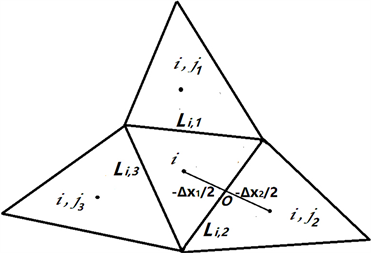(a)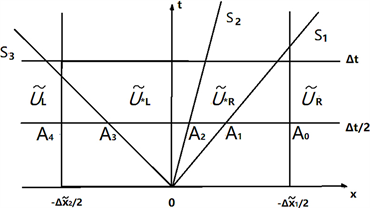(b)

Figure 1. The structure of approximate solution of Riemann problem

${\stackrel{˜}{U}}_{t}+F{\left(\stackrel{˜}{U}\right)}_{x}=0$

$\stackrel{˜}{U}\left(\stackrel{˜}{x},0\right)=\left\{\begin{array}{l}{\stackrel{˜}{U}}_{L}\text{\hspace{0.17em}}\text{\hspace{0.17em}}\text{\hspace{0.17em}}\stackrel{˜}{x}<0\\ {\stackrel{˜}{U}}_{R}\text{\hspace{0.17em}}\text{\hspace{0.17em}}\text{\hspace{0.17em}}\stackrel{˜}{x}>0\end{array}$

$T\left(\theta \right)=\left(\begin{array}{ccc}1& 0& 0\\ 0& \mathrm{cos}\theta & \mathrm{sin}\theta \\ 0& -\mathrm{sin}\theta & \mathrm{cos}\theta \end{array}\right)$ (4)

${U}_{i}^{n+1}={U}_{i}^{n}-\frac{\Delta t}{|{V}_{i}|}\underset{j=1}{\overset{3}{\sum }}{T}^{-1}\left({\theta }_{i,j}\right)F\left({\stackrel{˜}{U}}_{i,j}\right)|{L}_{i,j}|+\Delta t{S}_{i}$ (5)

${F}^{WAF}=\frac{1}{2}\left({c}_{4}{F}_{R}+{c}_{0}{F}_{L}\right)-\frac{1}{2}\underset{k=1}{\overset{N}{\sum }}sign\left({c}_{k}\right)\varphi \left({r}^{k},|{c}_{k}|\right)\Delta {F}^{k}$ (6)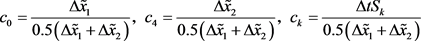(7)

${r}^{\left(k\right)}=\left\{\begin{array}{cc}\frac{{p}_{i}^{\left(k\right)}-{p}_{i-1}^{\left(k\right)}}{{p}_{i+1}^{\left(k\right)}-{p}_{i}^{\left(k\right)}}& {S}_{k}\ge 0\\ \frac{{p}_{i+2}^{\left(k\right)}-{p}_{i+1}^{\left(k\right)}}{{p}_{i+1}^{\left(k\right)}-{p}_{i}^{\left(k\right)}}& {S}_{k}<0\end{array}$ (8)

${\left[{F}^{hllc}\right]}_{\left(k\right)}={\left[{F}^{hll}\right]}_{\left(k\right)},k=1,2，\text{\hspace{0.17em}}\text{\hspace{0.17em}}\text{\hspace{0.17em}}{\left[{F}^{hllc}\right]}_{\left(3\right)}=\left\{\begin{array}{cc}{\left[{F}^{hll}\right]}_{\left(1\right)}{v}_{L}& {S}_{2}>0\\ {\left[{F}^{hll}\right]}_{\left(1\right)}{v}_{R}& {S}_{2}<0\end{array}$ (9)

${F}^{hll}=\left\{\begin{array}{ll}{F}_{L}\hfill & \text{\hspace{0.17em}}\text{\hspace{0.17em}}\text{\hspace{0.17em}}\text{\hspace{0.17em}}{S}_{1}\ge 0\hfill \\ \frac{{S}_{2}{F}_{L}-{S}_{1}{F}_{R}+{S}_{1}{S}_{2}\left({U}_{R}-{U}_{L}\right)}{{S}_{2}-{S}_{1}}\hfill & {S}_{1}<0<{S}_{2}\hfill \\ {F}_{R}\hfill & \text{\hspace{0.17em}}\text{\hspace{0.17em}}\text{\hspace{0.17em}}\text{\hspace{0.17em}}{S}_{2}\le 0\hfill \end{array}$ (10)

${S}_{1}=\mathrm{min}\left({\stackrel{˜}{u}}_{L}-\sqrt{g{h}_{L}},{\stackrel{˜}{u}}_{s}-\sqrt{g{h}_{s}}\right)，\text{\hspace{0.17em}}\text{\hspace{0.17em}}{S}_{3}=\mathrm{max}\left({\stackrel{˜}{u}}_{R}+\sqrt{g{h}_{R}},{\stackrel{˜}{u}}_{s}+\sqrt{g{h}_{s}}\right)$ (11)

${S}_{2}=\frac{{S}_{1}{h}_{R}\left({\stackrel{˜}{u}}_{R}-{S}_{3}\right)-{S}_{3}{h}_{L}\left({\stackrel{˜}{u}}_{L}-{S}_{1}\right)}{{h}_{R}\left({\stackrel{˜}{u}}_{R}-{S}_{3}\right)-{h}_{L}\left({\stackrel{˜}{u}}_{L}-{S}_{1}\right)}$ (12)

$\Delta t=CFL\underset{1\le i\le M}{\mathrm{min}}\underset{1\le j\le 3}{\mathrm{min}}\frac{\mathrm{min}\left(\Delta {\stackrel{˜}{x}}_{i,j,1},\Delta {\stackrel{˜}{x}}_{i,j,2}\right)}{\mathrm{max}\left({S}_{i,j,1},{S}_{i,j,3}\right)}$ (13)

${\iint }_{{V}_{i}}gH\nabla b\text{d}x\text{d}y=-gH\underset{j=1}{\overset{3}{\sum }}{\oint }_{{L}_{i,j}}\left({b}_{j},0\right)\cdot {n}_{j}\text{d}\zeta =-gH\underset{j=1}{\overset{3}{\sum }}{b}_{j}\cdot {n}_{j}|{L}_{i,j}|$

2.3. 计算条件

1) 计算区域：计算模型要求计算研究区域上下游需要由足够的计算长度，避免进出口条件设置的影响。结合现有河道地形资料，选取洪闸上游约4 km、下游约10 km，共计约14 km为计算区域。河道地形采用工程区附近2011年地形资料。

2) 网格剖分：考虑计算区域边界不规则形，边界条件复杂性，计算方法采用三角形剖分算法将物理区域划分为规则性三角形组成的计算区域，计算网格数为37,082个，为兼顾整个计算区域，同时为提高计算精度，在洪闸、分洪区、蓄洪区出口附近，采用了网格加密处理(如图2)。(a)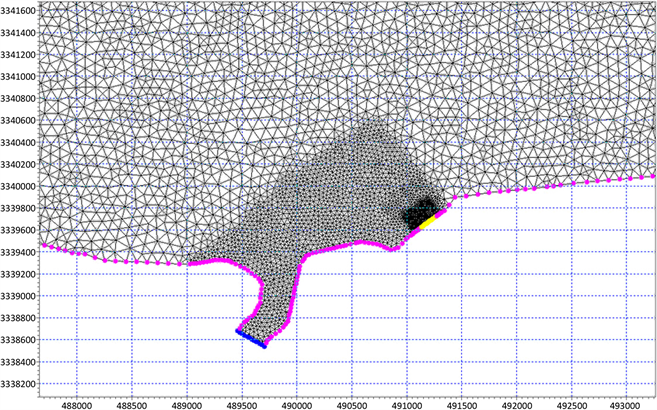(b)

Figure 2. Computational area mesh Generation and Local encryption Generation Diagram

2.4. 模型率定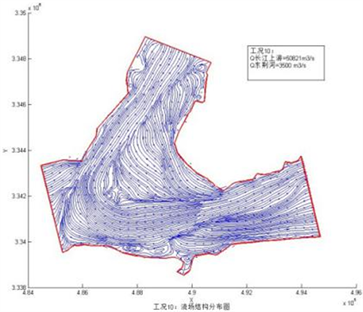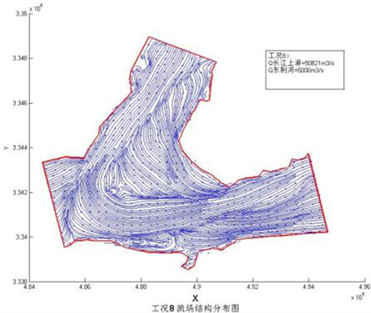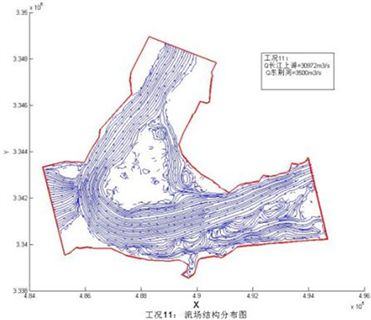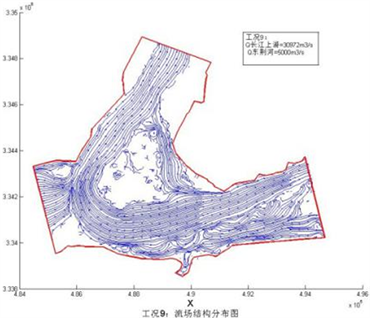Figure 3. Distribution of flow field

3. 数值计算

3.1. 长江与分洪汇合流计算

3.2. 洪闸、长江和分流汇合洪水计算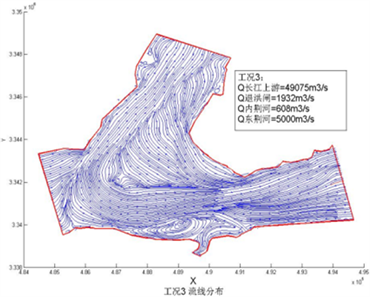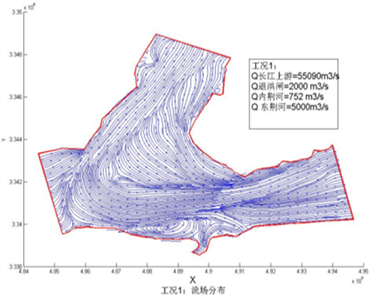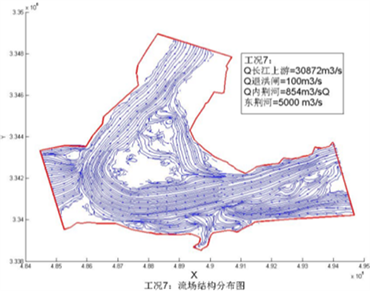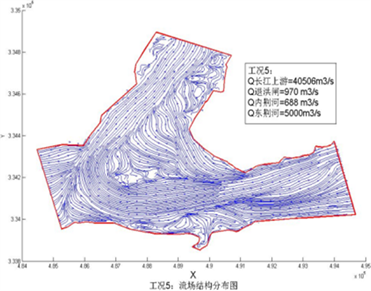Figure 4. Distribution of flow fieldTable 2. Characteristic value of flow velocity at monitoring points of embankment

4. 成果分析结论

1) 三股洪水同时汇合时，工程区域流场复杂，上游长江洪水同补元闸退洪洪水接触混合，受挤压偏向主槽，然后与内荆河排水混合，上游混合流在河道内进行短距离调整。在东荆河入口处，长江洪水再与东荆河分流洪水汇合，最后向右转弯，进入下游河道。

2) 通过流场分析，蓄洪区退洪过程一定程度上影响工程区长江洪水流场分布。通过不同工况数值计算，各监测点计算流速如图5。各工况测点流速大部分为0.05~0.3 m/s之间，在洪水相对较小时，堤防沿程各测点流速变化不大，长江洪水大于45,900 m3/s的工况时，在洪闸左侧长江下游堤段范围流速增加，最大值为0.31 m/s，结合流场分析，主要由于该区域为内荆河入江口处，同时叠加河道回流影响。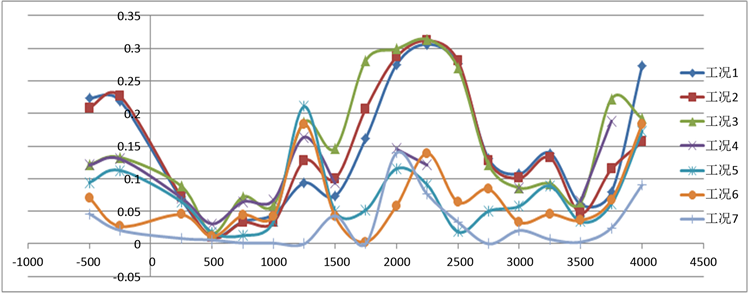Figure 5. Flow velocity distribution of embankment toe in lower reaches of Yangtze River

4. 结论

1) 蓄洪区退洪、长江行洪、东荆河分洪三股洪水汇合处流场结构非常复杂，在洪闸左侧长江下游河道出现两处较大范围的回流现象，但整体回流过程比较平稳，未出现主流偏离或主流折冲现象，整体布置比较安全。

2) 东荆河分流洪水同长江上游洪水汇合，主流偏左岸，然后流向下游，未直接影响长江上游区域，对补元闸工程、长江上游堤防影响不大。

3) 蓄洪区退洪与长江汇合流场复杂，工程附近流速较小，闸左、右两侧流速v < 0.4 m/s。蓄洪区退洪对工程附近长江下游流态有一定影响，增强了闸后长江下游堤防附近的回流强度。

 Garcia, R. and Kahawita, R.A. (1989) Numerical Solution of the St. Venant Equations with MacCormack Finite Difference Scheme. International Journal for Numerical Methods in Fluids, 6, 507-527.

 Akanbi, A.A. and Katopodes, N.D. (1988) Model for Flood Propagation on Initially Dry Land. Journal of Hydraulic Engineering, 114, 689-706.
https://doi.org/10.1061/(ASCE)0733-9429(1988)114:7(689)

 Cockburn, B. and Shu, C.W. (1989) TVB Runge-Kutta Local Projection Discontinuous Galerkin Finite Element Method for Scalar Conversation Laws II: General Framework. Mathematics of Computation, 52, 411-435.
https://doi.org/10.2307/2008474

 Cockburn, B., Lin, S.Y. and Shu, C.W. (1989) TVB Runge-Kutta Local Projection Dis-continuous Galerkin Finite Element Method for Scalar Conversation Laws III: One Dimensional Systems. Journal of Computational Physics, 84, 90-113.
https://doi.org/10.1016/0021-9991(89)90183-6

 Chen, G. and Noelle, S. (2017) A New Hydrostatic Reconstruction Scheme Based on Subcell Reconstructions. SIAM Journal on Numerical Analysis, 55, 758-784.
https://doi.org/10.1137/15M1053074

 Noelle, S., Pankratz, N., Puppo, G., et al. (2006) Well-Balanced Finite Volume Schemes of Arbitrary Order of Accuracy for Shallow Water Flows. Journal of Computational Physics, 213, 474-499.
https://doi.org/10.1016/j.jcp.2005.08.019

 胡四一, 谭维炎. 用TVD格式预测溃坝洪水波的演进[J]. 水利学报, 1989(7): 1-11.

 Bollermann, A., Chen, G., Kurganov, A., et al. (2013) A Well-Balanced Reconstruction of Wet/Dry Fronts for the Shallow Water Equations. Journal of Scientific Computing, 56, 267-290.
https://doi.org/10.1007/s10915-012-9677-5

 梁爱国, 槐文信, 赵明登. 用混合有限分析法求解二维溃坝洪水波的演进[J]. 水利水电技术, 2005, 36(10): 34-37.

 Alcrudo, F. and Garcia, N.P. (1993) A High-Resolution Godunov-Type Scheme in Finite Volumes for the 2D Shallow Water Equation. International Journal for Numerical Methods in Fluids, 16, 489-505.
https://doi.org/10.1002/fld.1650160604

 Harten, A., Engquist, B., Osherand, S., et al. (1987) A Uniformly High Order Ac-curacy Essentially Non-Oscillatory Schemes III. Journal of Computational and Applied Mathematics, 71, 231-303.
https://doi.org/10.1016/0021-9991(87)90031-3

 杨金波, 李订芳, 陈华. 和谐WAF格式在带干河床浅水波方程中的应用[J]. 华中科技大学学报(自然科学版), 2012, 40(2): 54-57+61.

 Toro, E.F. (1992) Riemann Problems and the WAF Method for Solving the Two-Dimensional Shallow Water Equations. Philosophical Transactions of the Royal Society of London. Series A, 338, 43-38.
https://doi.org/10.1098/rsta.1992.0002

 杨金波. WAF和MUSCL-HLLC有限体积格式及其在溃坝问题中的应用[D]: [博士学位论文]. 武汉: 武汉大学, 2011: 45-59.

Top